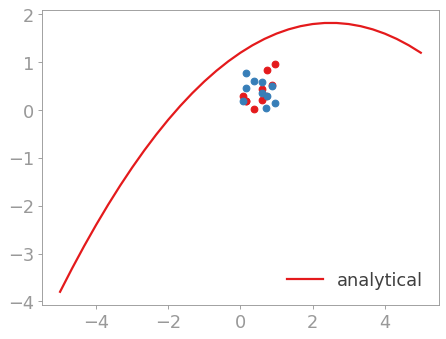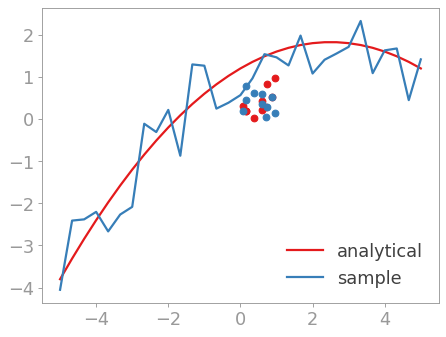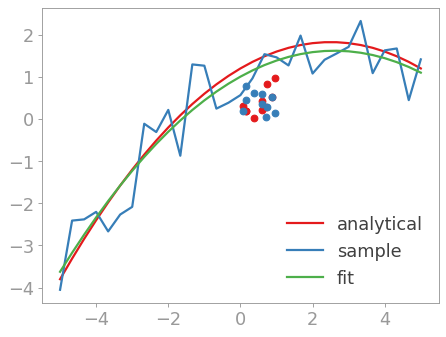# 拟合曲线¶

```>>> import scipy.optimize
```

```>>> def parabola(x, a, b, c):
...     return a*x**2 + b*x + c
...
```

```>>> params = [-0.1, 0.5, 1.2]
>>> x = np.linspace(-5, 5, 31)
>>> y = parabola(x, params, params, params)
>>> plt.plot(x, y, label='analytical')
[<matplotlib.lines.Line2D object at ...>]
>>> plt.legend(loc='lower right')
<matplotlib.legend.Legend object at ...>
>>> plt.show()
``````>>> r = np.random.RandomState(42)
>>> y_with_errors = y + r.uniform(-1, 1, y.size)
>>> plt.plot(x, y_with_errors, label='sample')
[<matplotlib.lines.Line2D object at ...>]
>>> plt.legend(loc='lower right')
<matplotlib.legend.Legend object at ...>
>>> plt.show()
``````>>> fit_params, pcov = scipy.optimize.curve_fit(parabola, x, y_with_errors)
```

```>>> for param, fit_param in zip(params, fit_params):
...     print(param, fit_param)
...
-0.1 -0.0906682795944
0.5 0.472361903203
1.2 1.00514576223
>>> y_fit = parabola(x, *fit_params)
>>> plt.plot(x, y_fit, label='fit')
[<matplotlib.lines.Line2D object at ...>]
>>> plt.legend(loc='lower right')
<matplotlib.legend.Legend object at ...>
>>> plt.show()
``````>>> print(pcov)
[[  1.64209005e-04   1.75357845e-12  -1.45963560e-03]
[  1.75357845e-12   1.16405938e-03  -1.73642112e-11]
[ -1.45963560e-03  -1.73642112e-11   2.33217333e-02]]
```

```>>> print(np.sqrt(np.diag(pcov)))
[ 0.01281441  0.03411831  0.15271455]
```